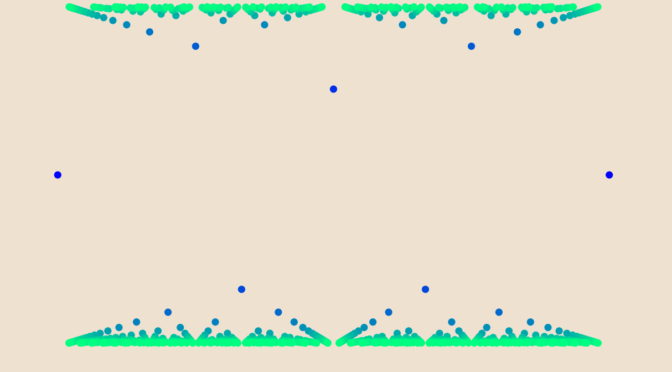Bounded functions and infimum, supremum

According to the extreme value theorem, a continuous real-valued function $$f$$ in the closed and bounded interval $$[a,b]$$ must attain a maximum and a minimum, each at least once.

Let’s see what can happen for non-continuous functions. We consider below maps defined on $$[0,1]$$.

First let’s look at $f(x)=\begin{cases} x &\text{ if } x \in (0,1)\\ 1/2 &\text{otherwise} \end{cases}$ $$f$$ is bounded on $$[0,1]$$, continuous on the interval $$(0,1)$$ but neither at $$0$$ nor at $$1$$. The infimum of $$f$$ is $$0$$, its supremum $$1$$, and $$f$$ doesn’t attain those values. However, for $$0 < a < b < 1$$, $$f$$ attains its supremum and infimum on $$[a,b]$$ as $$f$$ is continuous on this interval.

Bounded function that doesn’t attain its infimum and supremum on all $$[a,b] \subseteq [0,1]$$

The function $$g$$ defined on $$[0,1]$$ by $g(x)=\begin{cases} 0 & \text{ if } x \notin \mathbb Q \text{ or if } x = 0\\ \frac{(-1)^q (q-1)}{q} & \text{ if } x = \frac{p}{q} \neq 0 \text{, with } p, q \text{ relatively prime} \end{cases}$ is bounded, as for $$x \in \mathbb Q \cap [0,1]$$ we have $\left\vert g(x) \right\vert < 1.$ Hence $$g$$ takes values in the interval $$[-1,1]$$. We prove that the infimum of $$g$$ is $$-1$$ and its supremum $$1$$ on all intervals $$[a,b]$$ with $$0 < a < b <1$$. Consider $$\varepsilon > 0$$ and an odd prime $$q$$ such that $q > \max(\frac{1}{\varepsilon}, \frac{1}{b-a}).$ This is possible as there are infinitely many prime numbers. By the pigeonhole principle and as $$0 < \frac{1}{q} < b-a$$, there exists a natural number $$p$$ such that $$\frac{p}{q} \in (a,b)$$. We have $-1 < g \left(\frac{p}{q} \right) = \frac{(-1)^q (q-1)}{q} = - \frac{q-1}{q} <-1 +\varepsilon$ as $$q$$ is supposed to be an odd prime with $$q > \frac{1}{\varepsilon}$$. This proves that the infimum of $$g$$ is $$-1$$. By similar arguments, one can prove that the supremum of $$g$$ on $$[a,b]$$ is $$1$$.